COMPLETED QUANTUM FIELD THEORY (CQFT)

Nimit Theeraleekul B. Eng. (Electrical Communication)

(20 Aug. 2020)

Abstract. Quantum field theory is the basic foundation of the Standard model of particle physics, in which it is today most successful theory in prediction about the existence of Higgs particles. However, the crucial problem is about the field concept itself which is so weird that no one could understand how it is something like that! Armed with the new concept of Vacuum mechanics (mechanism of vacuum medium), then we could understand why and how it is be so, and then could improve it to a more complete quantum field theory!

Content: 1) Introduction. 1.1) Background. 1.2) What is the quantum field? 2) Relativistic quantum mechanics. 2.1) Problem in relativistic quantum mechanics. 2.2) What is the negative energy? 3) Problem in quantum field theory. 4) Should we bring back the aether? 4.1) Completed Einstein special theory of relativity (CSTR revisited.) 4.2) Completed quantum mechanical theory (CQMT revisited.) 5) Completed quantum field theory (CQFT). 6) What really quantum field is? 7) What is the Lorentz invariance in relativistic dynamics is? 7.1) Conventional derivation of Einstein mass energy equivalence. 7.2) Improve Einstein idea for mass energy equivalence. 7.3) New derivation for the increasing mass of a moving object. 7.4) Physical meaning of the Lorentz invariance. 8) Physical meaning of the negative energy. 9) Conclusion & discussion. 10) References.

1) Introduction. Anyone who familiar with Quantum field theory (QFT) would know that it is the basic foundation for all sectors of the Standard model of particle physics theory (SPT), which include electromagnetic (QED), weak (QFD) and strong (QCD) interactions into a single formula. However the famous SPT was criticized as an extraordinary ad hoc and ugly theory that it was clearly nonsense” . So what we really need is some fundamental new principle, and the best place to look for is from the theory’s weak points .

In the author opinion, the problem of SPT seems come from the incomplete concept of the QFT which is the main tool for SPT, and later we would found that it is indeed the problem was arise because we do not know – what really the quantum field is! Understanding the field concept and its working mechanism would pave the way to improve the more complete theory – Completed quantum field theory (CQFT) which is our main task of this paper.

1.1) Background. This article is the fruitful product extended from the author’s of the original article “Vacuum mechanics a New Approach to the Theory of Everything” (VMTE) .There are four most important theories involved in VMTE; Completed Maxwell electromagnetic field theory (CEMT), Completed Einstein special theory of relativity (CSTR), Completed Einstein general theory of relativity (CGTR) and Completed Quantum mechanical theory (CQMT).

In VMTE we have started with the fundamental hypothesis that “Vacuum medium is the fabric structure of vacuum space, and following is with the consequence hypothesis “Electrons and proton are tiny black holes (of vacuum medium)”. We have proved the existence of vacuum medium space instead of the conventional empty vacuum space, also showing how “condensed” vacuum medium were formed to be electrons and protons; the tiny black holes which are the origin of all the material matters existed in the universe!

Also we had shown that “vacuum mechanics” (i.e. the mechanism of vacuum medium) which is the action of vacuum medium or of condensed vacuum medium and the interaction between them, is responsible for all natural phenomena. Then we have used it for explaining the unsolved philosophic problems in EMT, STR and GTR and QMT.

Here are what which involved in improving QFT is by using CSTR and CQMT, and below is the summary of what it was done.

In CSTR we have improved the conventional STR to a more complete theory by adding it with a physical mechanism – vacuum medium to the conventional empty vacuum space; then it could solve the unexplained – relativistic effect such as increasing mass, length contraction and time dilation etc (please see detail in CSTR).

Lastly in CQMT we have improved the conventional concept of quantum mechanics with an understandable working mechanism of vacuum medium space which we called it as “quantum vacuum mechanics”. And what follows are that we could explain what which seems mysterious phenomena such as what the particle wave, wave is, showing how the de Broglie wave formula was derived, and also permits us to derive an understandable Schrödinger equation etc. (please see detail in CQMT).

1.2) What is quantum field theory? Now let us return to the story, first we will ask - what the quantum field theory is about, and here is one explanation;

In summary we could say that quantum field theory is a set of ideas and tools that combines three of the major themes of modern physics: the quantum theory, the field concept, and the principle of relativity .

This seems okay because all the readers who are familiar with both the conventional quantum mechanical theory (QMT) and the special theory of relativity (STR), would know how great they are. Then how could there is any problem occur as was claimed by the author. Maybe what which seems to be the problem was due to the unfamiliar field concept - quantum field in the quantum field theory itself in which it is very weird and we will talk in detail later.

2) Relativistic quantum mechanics. Before talking about the problem in QFT, what we should do first is looking back to see how QFT was arisen (via the relativistic quantum mechanics) and what the problems have been overcome. Below is a brief summary.

We know that nonrelativistic quantum mechanics was used in low energy regime in which the particle’s speed is low compare to light, such as stream of moving electrons which give rise to electrons waves used for electron microscope.  But when working in a high energy realm, such as using it as a tool in order to probe with sub atomic particles, we have to compliant with Einstein special theory; that is - a relativistic quantum mechanics was needed.

2.1) Problem in relativistic quantum mechanics. Historically, in developing a relativistic quantum mechanics we are facing with the most well-known crucial problem, i.e. the problem about negative energy. It is interesting to note that even it was told that negative energy is the important problem, but we still could not understand what it is! Maybe if we know what the negative energy is, then it could help to solve the entire problem involved; and finally it would pave the way turning the magic quantum field to a more realistic field – a more completed quantum field - we desired.

2.2) What is the negative energy? First let us see how the negative energy was interpreted. According to the well known Dirac “Hole theory” which regard vacuum as a “sea” in which all negative energy levels are occupied. Then because of the Pauli Exclusion Principle, electron cannot fall down to negative levels and thus become stable. When energy greater than twice the electron mass is given to the vacuum, an electron in the negative sea is excited to a positive energy level and a hole is created in the sea (Fig. 1a).

When an electric field is applied, a negative energy electron next to the hole moves in to fill the hole and creates another hole where it was before (Fig. 1b). The process is repeated and the net effect is that the hole moves in the opposite direction to the electron. So the hole is an antiparticle (positron) in which it behave as an ordinary particle with positive energy, but having charge, momentum and spin component opposite to the electron!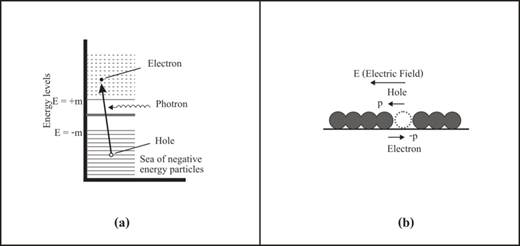Figure 1. Hole theory Diagram.

Thus Dirac has predicted the existence of the antiparticle and that pair of creation of a particle and antiparticle from vacuum will result if enough energy is injected. Anyway the hole theory cannot applied to boson in which the Pauli Exclusion Principle does not applied, Feynman and Stuckelburg abandon the idea of negative energy sea and applied the concept of backward traveling to the negative energy particle and which is equivalent to a moving forward positive energy antiparticle as show in Fig. 2.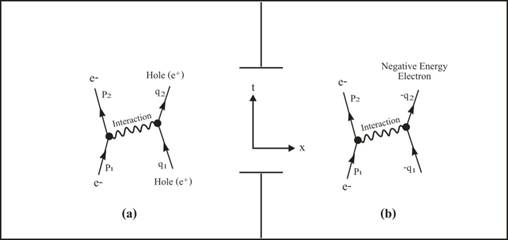Figure 2. Negative energy Interpretation.

What we have learned is, whether a sea of negative energy or backward travel in time is considered, a negative energy state force us to go from a one-particle description to a multiparticle world. A backward travelling particle induces vacuum fluctuation. The vacuum is no longer a static state, but rather like boiling water, constantly creating and annihilating pair of particles and antiparticles.

Up to now it seems that even we know something about the negative energy; but actually we could not understand what it is and how it gives rise to the antiparticles. Anyway, we will see that we could solve this problem by using vacuum mechanics (the mechanism of vacuum medium) concept, please wait.

3) Problem in quantum field theory. Now we are ready to consider in detail, what the problem in QFT is, by starting with Professor Zee words in the topic “Who needs quantum field theory?”  below.

“It is the peculiar confluence of QTM and STR that a new set of phenomena arise: Particle can be born and particle can die. It is matter of birth, life and death that requires the

development of a new subject in physics that of quantum field theory.”

“Let me give a heuristic discussion. In QTM the uncertainty principle tell us that the energy can fluctuate wildly over a small interval of time. According to STR, energy can be converted to mass and vice versa. With QTM and STR, the wildly fluctuate energy can metamorphose into mass, that is, into new particles not previous present.”

“…the whole subject of quantum field theory remains rooted in this harmonic paradigm…”

And, in the topic “From Mattress to Field” [5’] below.

“…the vacuum in quantum field theory is a stormy sea of quantum fluctuations…”

Consider the above words it sound good, but there is something which seems not okay;

a) Refer to the first and second paragraph, according to special theory of relativity, it only said that mass and energy is equivalence via the mass-energy relation; how that in high energy collision, particle-antiparticle pair could be created from input energy (and vice versa), in other words, what is its physical mechanism which can explain how it works?

And according to quantum mechanics, there is nothing dictates that - within the time interval according to the uncertainty principle, new particles could be created; in other words, what is its physical mechanism which can explain how it works?

b) According to the concept of STR and QMT, in which vacuum space is a blank space without anything that could provide physical mechanism for the above mentioned phenomena. To fulfill the lacking mechanism, quantum field idea was introduced as (mentioned in the third and forth paragraphs above). Anyway, to do so it gives rise to a more crucial problem i.e. where is the quantum field come from and how it is something like that?

Now before we consider in detail to see how and why the above problem of QFT was arisen, and then the quantum field was needed in order to solve the problem. First we have to go back to the conventional STR (in which it is one corner stone of the QFT) to see is there any problem and could we improve it, here below is the detail of the work.

4) Should we bring back the aether? Historically in October 1967, when the case against and for special relativity were being argued by Dingle and Mecrea in Nature, the editor of that journal summarized the discussion . Perhaps it was with genuine concern that he entitled his editorial ‘Don’t Bring Back the Ether’ . Up to now more than fifty years has pass, what we now think about the aether-should we bring it back?  For the author opinion, the answer is yes, of course, the reason is quite obvious today as concluded below.

a) Nowadays it is obvious that we can prove that there is something like the aether is existed; both by using an inexpensive simple experiment (by measuring the created magnetic field from two – solenoid set explained in VMTE) and by using the complicate and expensive experiment – the LHC at CERN which show how Higgs particles arisen from Higgs field that analogous to the aether !

b) According to Einstein quotation on May 5th 1920 at the University of Leyden which he accepted the aether (that formerly he rejected it), but there is no explanation why he changed his mind. For my opinion (the author), he tried to be a true physicist – not a magic physicist; i.e. because he could not explain how his conventional relativity theories work, so he want something acting as the mechanism for the physical phenomena involved (light wave

and gravity wave). Anyway, he could not do so, in which it was reflected from his proposed aether that have no any mechanical properties and no one know what it is!

c) Then now the problem is how could we turn Einstein’s aether that is an abnormal substance (which have no any mechanical properties, i.e. it is the same state of nothingness of vacuum in which it must have Lorentz invariance state of zero energy, zero momentum, zero angular momentum, zero charge, zero whatever [7’]) to a familiar normal substance while still keep the validity of the two relativity theories! Below is the way that shows how to do that.

4.1) Completed Einstein special theory of relativity (CSTR) revisited. First we have to look back to CSTR (mentioned in the introduction section) and would found that actually CSTR should be Einstein’s desired aether theory! More than that it is a better aether theory because the aether is not an imaginary strange medium, instead it is a real physical medium i.e. it is vacuum space medium.  So here we will summarize and concentrate about the mechanism of the medium which give rise to the relativistic effects as follow.

Below is the table (1) which shows how CSTR should be a better Einstein’s aether-based theory by comparing their feathers with STR and Newton law. Note: to understand how the comparison work, we have to refer to the light speed formula which is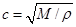(Where M = elasticity, ρ = mass density of medium) and the Lorentz transformation.

 Type Vacuum   space Light speed Relativistic effect Cause Kinematic Dynamics Classical      mechanics (Newton law) Empty ∞ No No --- Relativistic  mechanics      (STR) Empty C* (Refer. to any   initial frame) Time dilat. (#)     Length   Contract. (@) Mass  increase       (\$) #, @ - due to c*     Nothing for \$. Vacuum  mechanics    (CSTR) Vacuum  medium C (Refer. to vac.  med. frame) No Mass incr. Slow of mov. clock Resistive action   from vacuum       medium

Table 1

First, according to classical mechanics, because space is empty which mean ρ = 0, then we could say that c =. So the moving mass is constant because there is nothing to oppose its motion. This is corresponds to the concept of Galilean transformation in which Newton law is the same under its transformation!

Secondly, consider the conventional relativistic mechanics which is indeed very strange because its assumption is based on empty space while speed of light is still being c! And what follow from the Lorentz transformation give rise to the time dilation and length contraction. Also while there is nothing to resist the moving mass, but the moving mass still increasing its mass (as if it was subject to some resistance) without any cause!

Thirdly, according to the Vacuum mechanics’ case, because space is not empty, so light speed is c (speed of light propagation in the medium ρ). Also the moving masses were subjected to the resistance of vacuum medium as mentioned early; this is a reasonable cause which make the masses appeared as if they are increased, also give rise to the time dilation!

Up to now we have show how the conventional STR was improved to be the CSTR which is better because it could solve all the philosophical problem of STR. And because STR is one corner stone of the QFT, so we expect that CSTR could also solve the problem of QFT too. Next we will show how to improve QTM which is another corner stone of the QFT and using it to improve the problem in QFT.

4.2) Completed quantum mechanics (CQTM) revisited. Next we also have to look at CQTM (mentioned in the introduction section) in which we would found that the conventional QTM was improved by using the concept of the existence of vacuum medium (as was done with STR). Where a particle such as electron moving through the medium, it will disturb and create an electron wave, in which it is reasonable and more realistic to say that, this is the wave nature of an electron particle (rather than the conventional wave-particle dual property in which no physical mechanism do the job). Now we are ready to create a better

QFT.

 Detail  Topic Vacuum   space Particle       size Particle  speed Mass increase Wave     Appear Cause Anti-  particle 1 Mech.world   1.1 Classical            mech.   1.2 Relativistic         mech. Empty Visible Low    ( << c) No - - - sub-   atomic Medium      ( < c) Yes - No - 2 Quan.world    2.1Quantum.           Mechanics   2.2Rela.Quan.          Mechanics    2.3Quantum               Field Empty sub-     atomic Medium      ( < c) - Yes No - sub-     atomic High     ( ≤  c) Yes Yes No Yes    (No -      cause) Field 3 Vac. World   3.1Vacuum       Mechanics   3.2Quan.Vac.         Mechanics   3.3Rela.Quan.       Vac. Mech.       3.4Quantum        Vac. Field Vacuum medium sub-    atomic Medium     ( < c) Yes - Yes - sub-    atomic Medium     ( < c) - Yes Yes - sub-    atomic High     ( ≤  c) Yes Yes Yes Yes    (With-      cause)

Table 2

5) Completed quantum field theory (CQFT). As mentioned early that QFT was arisen from the two corner stone theories i.e. STR and QTM. And up to now we have improved both STR and QTM to a more complete theories (CSTR, CQTM) by adding the concept of vacuum medium, then make both the theories to be a more realistic one, in which both of them are equipped with the physical mechanism which could explain how they work (via vacuum medium - not just by their assumptions). When CSTR and CQTM are used instead of STR and QTM in QFT, then what we got is a more complete quantum field theory – CQFT. Table 3 above is the summary and comparison of what we have talked early and below is some more explanation.

In mechanical world (1), 1.2 is STR in which its weak point was eliminated and replaced by CSTR in Vacuum world (3.1). While in quantum world (2) QTM, (2.1) was improved and replaced by CQTM (3.2) in Vacuum world (3), and Relativistic quantum, QFT (2.2, 2.3) were improved and replaced by Relativistic quantum vacuum (3.3) and CQFT (3.4) respectively.

6) What really the quantum field is!  Up to now we are ready to talk in detail how to solve the problem of the quantum field in the conventional quantum field theory, which is a collection of an infinite number of connected harmonics oscillators distributed continuously in space. We know it is just an assumption with the reason behind from the concept in quantum mechanics that particles such as electrons have wave property and wave packet of photons acting like particles, in which we know that there is no explanation where the field come from and how they are so.

Now armed with our idea which we have said that every unexplained phenomenon occurs in relativity and quantum mechanics could be solved by using the mechanism of vacuum medium. In VMTE mentioned early, the author has giving its properties without any physical intuition picture. Here we will follow the idea which illustrate by Professor Yorikiyo Nagashima , by using the mechanical model of crystal lattice in metal that is a familiar phenomenon in condensed matter physics. In the diag. fig 3. below is a mechanical model of point masses connected by springs, which show positive ionize atoms that distributed regularly lattices wise – this is what the field made of!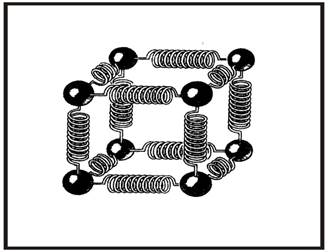Figure 3. Mechanical oscillator model of a field.

To apply the above model of the field to our vacuum medium, we have to imagine that each sphere of point mass is an infinitesimal part of the medium connects (attracts) with the neighboring parts around via their own gravity, forming to be the continuum of the medium space (explained detail in VMTE). This gives us the way how to visualize vacuum medium concept!

Next (as explained detail in CQMT) when particle such as an electron moving (with constant speed) it disturbs and created a co–moving standing wave called electron wave, this resemble to the harmonics oscillator in the quantum field. Also when the electron oscillates (for a short period) it will generate an outgoing wave packet called photon, but if the oscillation is continuous, the outgoing wave will also radiate with continuous wave too. For the reason of the mechanism explained, it is no need to make the assumption that there is the weird quantum field permeates in all vacuum space!

Finally let us starting with Professor Zee words in the topic “From Mattress to Field” [5’] below.

“We start with a mattress for pedagogical reasons. Of course nobody believes that the fields observed in Nature, such as the meson field or the photon field, are actually constructed of point masses, tied together with springs. The modern view, which I will call Landau-Ginzburg, is that we start with the desired symmetry…”

From the above wording we could see historically how quantum field was developed i.e. via the mattress idea which constructed of point masses and tied together with springs, it is something resemble the idea which we just talking above. Anyway at that time, people who developed the idea cannot found something (like vacuum medium) to act as the physical mechanism, then they ignored the mattress idea and replaced with the mathematical idea in which we cannot understand how and why it is be so – this is the modern view, which give rise to the serious fundamental problems in QFT that is the conventional quantum field!

7) What is the Lorentz invariance in relativistic dynamics? For someone who has read CGTR paper would found that the author has talk about physical meaning of the Lorentz invariance in relativistic kinematics there. Here for high energy collision in QFT, Lorentz invariance is very important, but we still could not understand its working mechanism or its physical meaning! So below we will show how the CQFT could explain this mysterious Lorentz invariance.

We know that the Lorentz invariance is the relation between total energy, momentum and rest mass as in equation (1) below. And we also know that it was derived from Einstein mass energy equivalence relation in (2), together with the assumption about the increasing mass (3) and the momentum (4) of the moving object respectively, which also just mathematical assumption!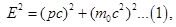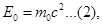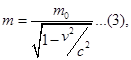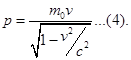So if we know the physical meaning of equation (2), (3) and (4) then we could understand the Lorentz invariance too. To do so first of all we will have to see how Einstein derived this most famous equation (2) in the conventional STR by his thought experiment  as below.

7.1) Conventional derivation of Einstein mass energy equivalence. According to the diagram below, when light particle (with energy E which also carry momentum p by the relation E = pc), emitted (with velocity c) from the left side of the box (which mount on free wheels) with mass M. So the box recoils with a momentum -E/c and then moving to the left with velocity v = - E/Mc.

Suppose that M is so large that v is so small, v << c and the box motion is well described by Newtonian non-relativistic concept. When the light move to right it was absorbed there, and while the moving box stop at time Δt = L/c, (L is the box’s length). So it correspond to the box move with the distance ΔX = vΔt = - E/Mc .L/c.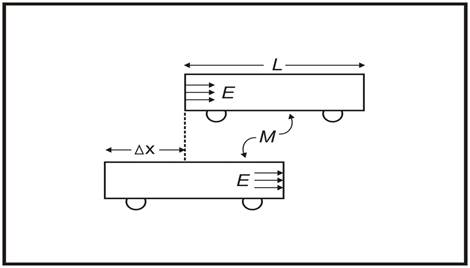Figure 4. Einstein thought experiment for mass energy equivalence.

The reason that the box stop after moving, because it try to keep the center of mass fix. This means the deposited energy of the moving light equal to some mass, m, i.e.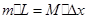or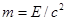, (as equation (2)) which mean that mass equivalence to energy over the square speed of light!

7.2) Improve Einstein idea for mass energy equivalence! First we will treat Einstein thought experiment as a relativistic concept one (even through v << c), then from the experiment that the moving box is stop at time Δt = L/c, now it will change to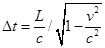! For someone who familiar with STR will remember that, this new time was interpreted as time dilation effect due to Einstein concept that - light speed c in the box is constant. But here we have use our new concept - light speed c is constant reference to vacuum medium in which it give us the same “new time” equation while having different meaning (please see detail explanation in CSTR).

Next when we use the new time Δt to do the same thing as in the Einstein’s derivation, then what we will get here is a new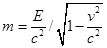. But if we change the moving box to be a stationary one, i.e. v = 0, then what we got is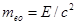, while we will call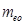as the “equivalence mass” of the moving energy of the light in the stationary box!

And in order to make it clear about the symbol m, then we have to rewrite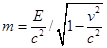, as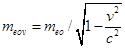in which we will call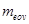as the “equivalence mass” of the moving energy of the light in the moving box!

Finally we come to the important question – physically, how is the increasing energy of the moving light occurring? Armed with the existing of vacuum medium, it is not difficult to see that the reason is because the moving time of the moving light in the box is increased. In other words, we may say that the increasing energy of the moving light was due to the dynamics action of the vacuum medium!

7.3) New derivation for the increasing mass of the moving object. Reader who have read CSTR paper would found that the author had derive how the moving object increase its mass by using the Lienard-Wiechert potential formula. Here we will use Einstein thought experiment about mass energy equivalence for starting point as follow.

First let us return to the equation., in Einstein thought experiment (sec. 7.1), and when we replace the result from our modified experiment (sec. 7.2), which is,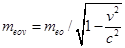, or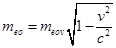, then we will get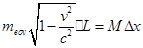. Next we can rearrange it as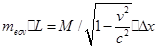in which we can see that while the equivalence mass of light in the left hand side has increased i.e.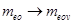, the mass M in the right hand side has  also increased i.e.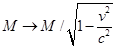due to the dynamics action of the vacuum medium too!

Note that the mass M has assigned as the “proper” mass, then we should redefine it as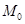and also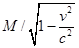should be rewrite as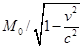.

Finally we can say that in the moving system, the mass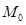will increase its mass as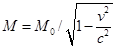which is the relativistic mass M! Lastly, in order to make the symbol of mass M and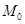to be the same as was assigned before i.e. m and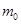in eq. (3), then we will write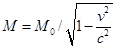as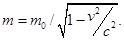7.4) Physical meaning of the Lorentz invariance. Now we will consider it as follow;

a) First let us consider equation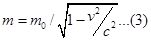again, and then rearrange it as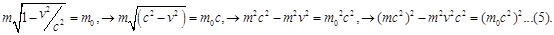Finally when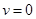what we get is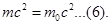Next, from eq. (5) if we replace v with p/m, then what we get is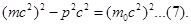b) We know that the energy of the moving mass is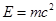and if we substitute it in eq. (6) and (7), then finally we will get the rest energy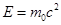(which is constant) and the Lorentz invariance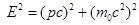in which it is the total energy that increasing with the velocity of the moving mass.

c) But when looking back to sec. 7.3, we see that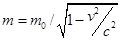(which is the moving increased mass from its rest mass) was due to the dynamics action of the vacuum medium. And because the Lorentz invariance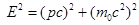was derived fromtoo, then we may conclude that the increasing energy of the moving particle (over its rest energy) is due to the dynamics action of the vacuum medium!

8) Physical meaning of the negative energy. We have talk about negative energy early and found that no one know what it is, but now we have the new idea that vacuum space is not an empty one, instead it is vacuum medium space! Here we will show that actually it is vacuum medium which manifest as the mysterious negative energy; below how vacuum medium do the job of the negative energy.

a) According to Dirac Hole theory which regard vacuum as a “sea” of negative energy in which it act as the source of particle – antiparticle pair production. Anyway, we know that this contradict to vacuum space concept in the conventional STR (i.e. space is empty), so how could they solve it?

b) It seems that in order to obey the empty vacuum concept of STR, so Feynman and Stuckelberg abandoned the idea of the negative energy sea and only applied the concept of backward travelling to the negative energy particle and then interpreted it as antiparticle (with positive energy) travel forward in time, but how?

c) In summary Feynman’s antiparticle idea seem arise from handle the problem of the advance solution of Maxwell equation according to Wheeler – Feynman absorber theory . The idea is that when charge particle such as electron oscillate then create retarded waves while at the same time others charges react back advance solution, and the crucial point is that the interaction of the original charges with the others is haft through the advanced haft through the retarded waves then the advanced waves could be eliminated! Below is mathematical manipulation which involved in the process mentioned.

The total output energy,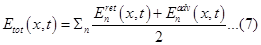Then if the free energy,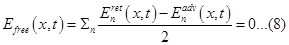Finally what we got is that the total output energy is equal to the retarded waves as (9).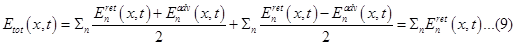d) To visualize how the above idea work, Let us refer to a simple audio system show in dia. Fig. 6; first start with the loudspeaker’s drum which is vibrated and making a push – pull back and forth to the air in front, creating series of oscillation (between inertial force and elastic restoring force) which manifest as traveling wave moving forward, finally making vibration of the eardrum.

e) Next consider the flowing energy involved in the working process of the system, by starting with input energy (from loudspeaker’s drum) which then transfers via wave motion (by the coupled motion of an extended array of oscillators) as the output energy to the eardrum (without any energy loss in an ideal case). In which we could write it as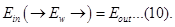where we can see that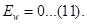f) But remember that the equation of each oscillator motion is given by the dynamic balance between the kinetic (energy) force on the particle at any instant and the elastic potential (energy) force, so we could write it as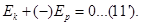Or we could write it as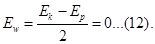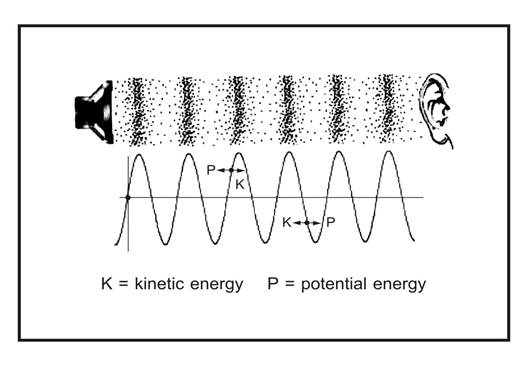Figure5. Negative energy waves’ mechanism.

g) Now let us return to the total energy of each one cycle of each one simple harmonic oscillation process where the total energy is haft the sum of the kinetic energy and the potential energy i.e.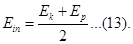And if we add (13) to (12) we get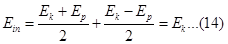h) Next before to visualize mathematical formulas (7), (8) and (9) by compare them to (12) (13) and (14), we have to rewrite them in simple one dimension form as (7’), (8’) and (9’) below.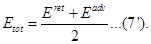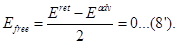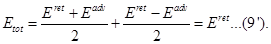i) By comparing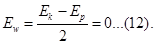to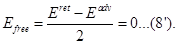we see that they have the same form. Next we know that the wave energy of air medium (12) is zero according to (10) and (11) in (e), and this corresponds to the unknown free field energy (8’). But if we accept that vacuum space is not empty, instead it is vacuum medium space, then we could understand that actually it is wave energy of vacuum medium!

j) Next we will consider how to relate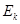to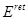and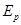to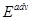. first let us refer to the simple audio system show in dia. Fig. 6 as explain in (f) again, here we consider that at each points of the sine wave curve solution which was got from equation of motion, we see thatBut the important point here is that we could interpret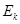as forward energy part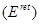while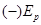could interpret as backward energy part i.e.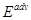.

k) Now we come back to the comparison between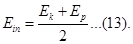to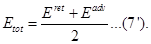and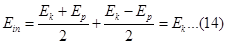toIn the first row it is not difficult to see that why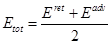in (7’) when relate it to (13) which is the total energy explained in (g). And in the second row if we add the wave energy (12) in the left side of (13) and (8’) in the right side of (7’), then both side have the same meaning i.e.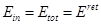!

m) Finally we will go back to consider about the negative energy,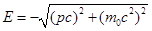(which is one of the two solutions of the energy-momentum relation (2)). And we know that the energy-momentum was arisen from the concept of the dynamics action of vacuum medium space mentioned in section 7.3. Together with the interpretation thatas positive energy partwhilecould interpret as negative energy part i.e.mentioned in (j). Then it is reasonable to interpret thatis the positive energy andis the negative energy of the relativistic particle!

10) Conclusion & discussion. Here we will comment something about what we have talked and have left.

a) First of all, the author has to remind that this paper was written in a rather philosophical idea without mathematical rigor, in which the main purpose is to give us a new approach for the experts to work out further.

b) One of the most interesting points that the readers may found is that all the philosophical problems starting from the concept of STR which rule out the existing of something with act as the medium (such as aether) in vacuum space i.e. the concept of void space! And we have seen that QMT also base on the concept of void space, then it is not surprise that QFT have follow in using the same concept.

c) After some reading the readers may notice that the main idea which was introduced in this paper is to concentrate on the weak points in QFT that arisen from the concept of void space which lacking of any medium that provide their physical working mechanism for the weird QF concept!

d) The readers who familiar with QFT would know that it is a complicate theory, here in this paper, what we have talked about is only a small part of a large amount of the theory. Indeed once we know its foundation i.e. what the quantum field is, it is not so difficult for solving the remain problems!

11) References.

(Precaution; Several text books with different authors were used as the reference and each author used different pattern and different notation for the same formula. Here in this paper, the author has made some change of the original pattern and notation for the readers convenient, so please be careful!)

 Robert Oerter “The Theory of Almost Everything, the Unsung Triumph of Modern

Physics”, p.208.

 Gerard ‘tHooft, “In search of the ultimate building blocks” p. 127. For [4’] p.127-129.

 Nimit Theeraleekul, the articles now presenting in www.vacuum-mechanics.com.

 Michael E. Pesin and Daniel V. Shroeder, “Introduction to Quantum Field Theory”

p. xi.

 A. Zee “Quantum field theory in a nutshell” p.3-6. For [5’] topic …p.16-23, and for [5’’]

topic -The energy of the vacuum.p.64

 L. Marder “TIME AND THE SPACE-TRAVELLER”, p. 27.

 Tian Yu Cua, “Conceptual Development of 20th Century Field Theories”, topic Open

questions p.312. For [7’] topic Vacuum p.176.

 Yorikiyo Nagashima, “Elementary Particle Physics” volume 1: Quantum Field Theory

and Particles, topic Particles and Fields p. 28.

 John B. Kogut, “Introduction to Relativity” p. 65

 Wikipedia, the free ency…https://en.wikipedia.org/wiki/Wheeler-

Feynman_absorber_theory 15 June 2016.

……………………..